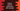# IntEnum in python explanation with example## IntEnum in python:

Using IntEnum(), we can create enumerated constants with subclass of int. This method is used to create enumeration based on integers in python.

In this tutorial, we will learn how to use IntEnum with example.

### Example of IntEnum:

Let’s take a look at the below example. Here, we are using normal enum :

``````from enum import Enum

class Days(Enum):
SUN = 1
MON = 2
TUES = 3

print(Days.SUN == 1)``````

Running this program will print False. We can’t compare a enum value with an integer. But if we use an IntEnum :

``````from enum import IntEnum

class Days(IntEnum):
SUN = 1
MON = 2
TUES = 3

print(Days.SUN == 1)``````

It prints True.

Now, check the below program:

``````from enum import IntEnum

class Days(IntEnum):
SUN = 1
MON = 2
TUES = 3

class Months(IntEnum):
JAN = 1
FEB = 2

print(Days.SUN == Months.JAN)``````

It prints True. Both Days and Months are two different Enum classes but since we are using IntEnum, both Days.SUN and Months.JAN gives the same value 1 and it prints True.